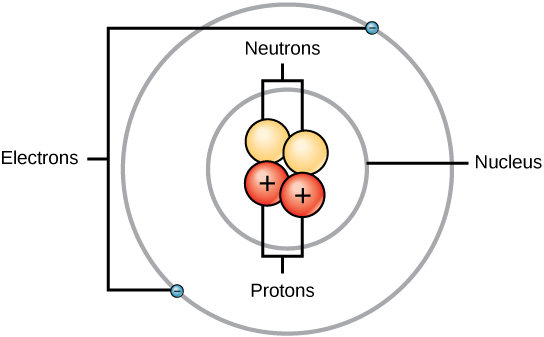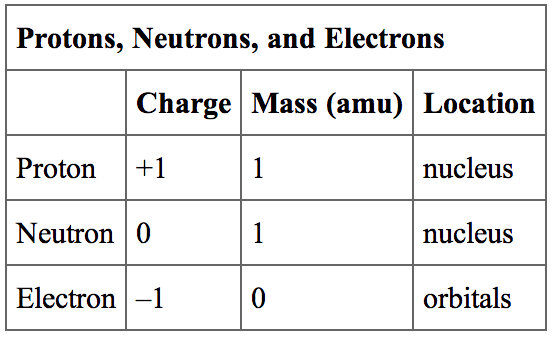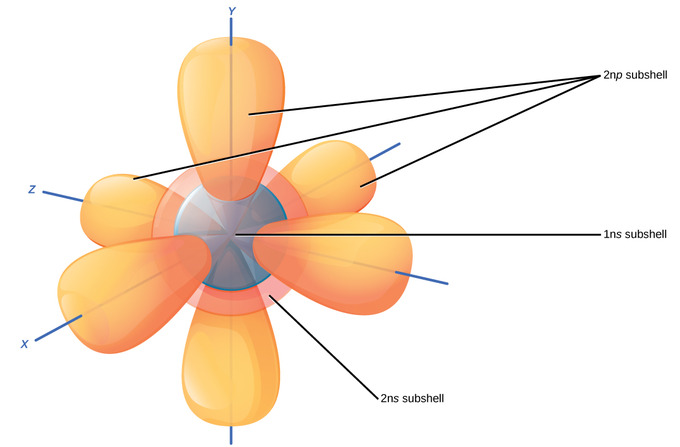## Overview of Atomic Structure

Atoms are made up of particles called protons, neutrons, and electrons, which are responsible for the mass and charge of atoms.

### Learning Objectives

Discuss the electronic and structural properties of an atom

### Key Takeaways

#### Key Points

• An atom is composed of two regions: the nucleus, which is in the center of the atom and contains protons and neutrons, and the outer region of the atom, which holds its electrons in orbit around the nucleus.
• Protons and neutrons have approximately the same mass, about 1.67 × 10-24 grams, which scientists define as one atomic mass unit (amu) or one Dalton.
• Each electron has a negative charge (-1) equal to the positive charge of a proton (+1).
• Neutrons are uncharged particles found within the nucleus.

#### Key Terms

• atom: The smallest possible amount of matter which still retains its identity as a chemical element, consisting of a nucleus surrounded by electrons.
• proton: Positively charged subatomic particle forming part of the nucleus of an atom and determining the atomic number of an element. It weighs 1 amu.
• neutron: A subatomic particle forming part of the nucleus of an atom. It has no charge. It is equal in mass to a proton or it weighs 1 amu.

An atom is the smallest unit of matter that retains all of the chemical properties of an element. Atoms combine to form molecules, which then interact to form solids, gases, or liquids. For example, water is composed of hydrogen and oxygen atoms that have combined to form water molecules. Many biological processes are devoted to breaking down molecules into their component atoms so they can be reassembled into a more useful molecule.

### Atomic Particles

Atoms consist of three basic particles: protons, electrons, and neutrons. The nucleus (center) of the atom contains the protons (positively charged) and the neutrons (no charge). The outermost regions of the atom are called electron shells and contain the electrons (negatively charged). Atoms have different properties based on the arrangement and number of their basic particles.

The hydrogen atom (H) contains only one proton, one electron, and no neutrons. This can be determined using the atomic number and the mass number of the element (see the concept on atomic numbers and mass numbers).Structure of an atom: Elements, such as helium, depicted here, are made up of atoms. Atoms are made up of protons and neutrons located within the nucleus, with electrons in orbitals surrounding the nucleus.

### Atomic Mass

Protons and neutrons have approximately the same mass, about 1.67 × 10-24 grams. Scientists define this amount of mass as one atomic mass unit (amu) or one Dalton. Although similar in mass, protons are positively charged, while neutrons have no charge. Therefore, the number of neutrons in an atom contributes significantly to its mass, but not to its charge.

Electrons are much smaller in mass than protons, weighing only 9.11 × 10-28 grams, or about 1/1800 of an atomic mass unit. Therefore, they do not contribute much to an element’s overall atomic mass. When considering atomic mass, it is customary to ignore the mass of any electrons and calculate the atom’s mass based on the number of protons and neutrons alone.

Electrons contribute greatly to the atom’s charge, as each electron has a negative charge equal to the positive charge of a proton. Scientists define these charges as “+1” and “-1. ” In an uncharged, neutral atom, the number of electrons orbiting the nucleus is equal to the number of protons inside the nucleus. In these atoms, the positive and negative charges cancel each other out, leading to an atom with no net charge.Protons, neutrons, and electrons: Both protons and neutrons have a mass of 1 amu and are found in the nucleus. However, protons have a charge of +1, and neutrons are uncharged. Electrons have a mass of approximately 0 amu, orbit the nucleus, and have a charge of -1.

Exploring Electron Properties: Compare the behavior of electrons to that of other charged particles to discover properties of electrons such as charge and mass.

### Volume of Atoms

Accounting for the sizes of protons, neutrons, and electrons, most of the volume of an atom—greater than 99 percent—is, in fact, empty space. Despite all this empty space, solid objects do not just pass through one another. The electrons that surround all atoms are negatively charged and cause atoms to repel one another, preventing atoms from occupying the same space. These intermolecular forces prevent you from falling through an object like your chair.

Interactive: Build an Atom: Build an atom out of protons, neutrons, and electrons, and see how the element, charge, and mass change. Then play a game to test your ideas!

## Electron Orbitals

Electron orbitals are three-dimensional representations of the space in which an electron is likely to be found.

### Learning Objectives

Distinguish between electron orbitals in the Bohr model versus the quantum mechanical orbitals

### Key Takeaways

#### Key Points

• The Bohr model of the atom does not accurately reflect how electrons are spatially distributed around the nucleus as they do not circle the nucleus like the earth orbits the sun.
• The electron orbitals are the result of mathematical equations from quantum mechanics known as wave functions and can predict within a certain level of probability where an electron might be at any given time.
• The number and type of orbitals increases with increasing atomic number, filling in various electron shells.
• The area where an electron is most likely to be found is called its orbital.

#### Key Terms

• electron shell: The collective states of all electrons in an atom having the same principal quantum number (visualized as an orbit in which the electrons move).
• orbital: A specification of the energy and probability density of an electron at any point in an atom or molecule.

Although useful to explain the reactivity and chemical bonding of certain elements, the Bohr model of the atom does not accurately reflect how electrons are spatially distributed surrounding the nucleus. They do not circle the nucleus like the earth orbits the sun, but are rather found in electron orbitals. These relatively complex shapes result from the fact that electrons behave not just like particles, but also like waves. Mathematical equations from quantum mechanics known as wave functions can predict within a certain level of probability where an electron might be at any given time. The area where an electron is most likely to be found is called its orbital.

### First Electron Shell

The closest orbital to the nucleus, called the 1s orbital, can hold up to two electrons. This orbital is equivalent to the innermost electron shell of the Bohr model of the atom. It is called the 1s orbital because it is spherical around the nucleus. The 1s orbital is always filled before any other orbital. Hydrogen has one electron; therefore, it has only one spot within the 1s orbital occupied. This is designated as 1s1, where the superscripted 1 refers to the one electron within the 1s orbital. Helium has two electrons; therefore, it can completely fill the 1s orbital with its two electrons. This is designated as 1s2, referring to the two electrons of helium in the 1s orbital. On the periodic table, hydrogen and helium are the only two elements in the first row (period); this is because they are the sole elements to have electrons only in their first shell, the 1s orbital.

### Second Electron ShellDiagram of the S and P orbitals: The s subshells are shaped like spheres. Both the 1n and 2n principal shells have an s orbital, but the size of the sphere is larger in the 2n orbital. Each sphere is a single orbital. p subshells are made up of three dumbbell-shaped orbitals. Principal shell 2n has a p subshell, but shell 1 does not.

The second electron shell may contain eight electrons. This shell contains another spherical s orbital and three “dumbbell” shaped p orbitals, each of which can hold two electrons. After the 1s orbital is filled, the second electron shell is filled, first filling its 2s orbital and then its three p orbitals. When filling the p orbitals, each takes a single electron; once each p orbital has an electron, a second may be added. Lithium (Li) contains three electrons that occupy the first and second shells. Two electrons fill the 1s orbital, and the third electron then fills the 2s orbital. Its electron configuration is 1s22s1. Neon (Ne), on the other hand, has a total of ten electrons: two are in its innermost 1s orbital, and eight fill its second shell (two each in the 2s and three p orbitals). Thus, it is an inert gas and energetically stable: it rarely forms a chemical bond with other atoms.

### Third Electron Shell

Larger elements have additional orbitals, making up the third electron shell. Subshells d and f have more complex shapes and contain five and seven orbitals, respectively. Principal shell 3n has s, p, and d subshells and can hold 18 electrons. Principal shell 4n has s, p, d, and f orbitals and can hold 32 electrons. Moving away from the nucleus, the number of electrons and orbitals found in the energy levels increases. Progressing from one atom to the next in the periodic table, the electron structure can be worked out by fitting an extra electron into the next available orbital. While the concepts of electron shells and orbitals are closely related, orbitals provide a more accurate depiction of the electron configuration of an atom because the orbital model specifies the different shapes and special orientations of all the places that electrons may occupy.